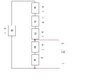# KVL / KCL (Circuit Analysis)

• Engineering
• carlodelmundo
In summary, the conversation discussed finding the voltage Vab in a circuit using KVL. The problem asked for Vab, but there was confusion about whether to include all resistances or just the potential difference between two points. After clarification, it was determined that Vab can be calculated by finding the potential difference between Va and Vb, and this can be done by using all resistances or just the voltage gains and drops in the loop.

## Homework Statement

Basically, find Vab

[PLAIN]http://carlodelmundo.com/hw/circuit.png [Broken]

Is this correct -- note: please tell me that my methodology is sound; DO NOT GIVE ME A NUMERICAL ANSWER.

The problem asks for VAB. What's weird to me is that there is a potential across two terminals? (The weird part is: the terminals are connected to another voltage source!)

I used KVL... and I'm fairly accurate that my calculation is correct. However, I am confused with finding the actual VAB. Can I simply apply Ohm's law, and "lump" all of the resistors into one resistor (composition?) Would VAB be equivalent to the sum of all the voltages (taking polarities into account)?

Last edited by a moderator:
Your current calculations look good, but I think Vab is wrong. Keep in mind Vab = Va - Vb i.e. the voltage between two different points. As you have done in the first part of your current equations, all the voltage 'gains' must equal all of the voltage 'drops' (Kirchoffs Voltage Law) in the loop, but Vab doesn't include all of the resistances in the loop.

The terminals can be disregarded also, since they merely represent a connection point, but with or without them the circuit is the same.

Check out this redrawn circuit.

#### Attachments

•Find Vab.JPG
7.1 KB · Views: 592
I see. Based on your chart, I may have gained some insight. Let me clarify-- so we're not supposed to use all of the resistances in the circuit?

Is it correct to assume that to calculate Vab, I may simply just find the potential difference between Va and Vb?

e.g.: according your chart, Vab = vr3 + V4v = 3(i) + 4 = 3(16/6) + 4 = 12V? If this is true, can I solve for the reverse and negate the answer? (the other resistors and other voltage source)

hi carlodelmundo!Zrynis offline, so I'll confirm that 12 V is correct …

you calculated it via the 4 V, but if you'd gone the other way, that will give the same result …

try it and see (you've probably already done so)!the whole point of KVL is that the potential differences (for want of a better phrase) all add to zero around a loop …

when you're asked for the potential between two points, it's exactly the same as the sum of the voltages you'd use in KVL itselfThanks! this makes a lot of sense now!